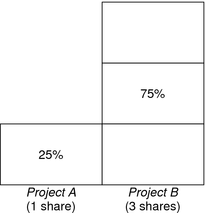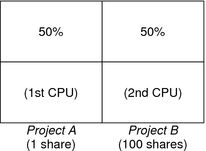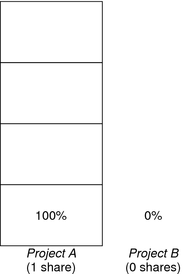System Administration Guide: Virtualization Using the Solaris Operating System

# CPU Share Examples

Assume you have a system with two CPUs running two parallel CPU-bound workloads called A and B, respectively. Each workload is running as a separate project. The projects have been configured so that project A is assigned SA shares, and project B is assigned SB shares.

On average, under the traditional TS scheduler, each of the workloads that is running on the system would be given the same amount of CPU resources. Each workload would get 50 percent of the system's capacity.

When run under the control of the FSS scheduler with SA=SB, these projects are also given approximately the same amounts of CPU resources. However, if the projects are given different numbers of shares, their CPU resource allocations are different.

The next three examples illustrate how shares work in different configurations. These examples show that shares are only mathematically accurate for representing the usage if demand meets or exceeds available resources.

## Example 1: Two CPU-Bound Processes in Each Project

If A and B each have two CPU-bound processes, and SA = 1 and SB = 3, then the total number of shares is 1 + 3 = 4. In this configuration, given sufficient CPU demand, projects A and B are allocated 25 percent and 75 percent of CPU resources, respectively.## Example 2: No Competition Between Projects

If A and B have only one CPU-bound process each, and SA = 1 and SB = 100, then the total number of shares is 101. Each project cannot use more than one CPU because each project has only one running process. Because no competition exists between projects for CPU resources in this configuration, projects A and B are each allocated 50 percent of all CPU resources. In this configuration, CPU share values are irrelevant. The projects' allocations would be the same (50/50), even if both projects were assigned zero shares.## Example 3: One Project Unable to Run

If A and B have two CPU-bound processes each, and project A is given 1 share and project B is given 0 shares, then project B is not allocated any CPU resources and project A is allocated all CPU resources. Processes in B always run at system priority 0, so they will never be able to run because processes in project A always have higher priorities.